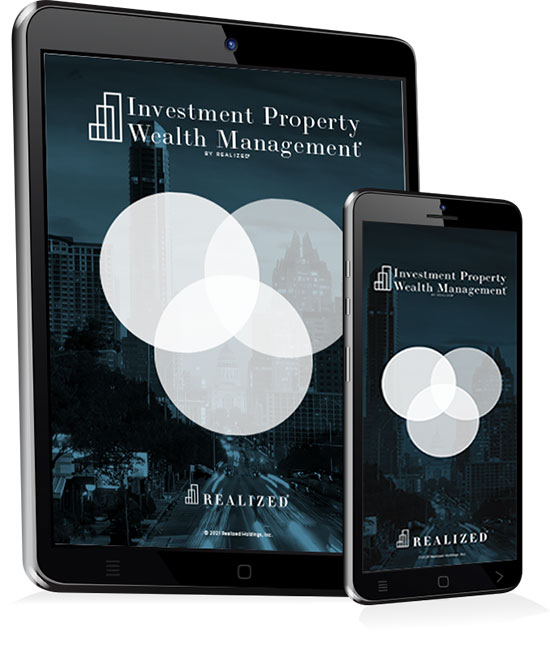Calculating the return on your real estate investment property is important to understand how well it is performing. There are a number of metrics used by real estate professionals to determine the performance of a property. In this article, we’ll start with some return on investment (ROI) basics and then go into calculations that get progressively more involved, as we factor in more components of return.

### Return On Investment (ROI)

Return on investment (ROI) measures the gain or loss since acquiring an investment or during some specific period of time. Either way, it is a measure of performance across a period of time.

The ROI calculation is a simple formula:

Net Return on Investment / Cost of the Investment * 100

Net Return is equal to (Current Value - Initial Value). Another way of stating ROI is:

(Current Value - Initial Value) / Cost of Investment * 100

As an example, let’s say we invest \$100,000 in a property. One year later, the property is worth \$104,000. The return for this property is:

104,000 - 100,000 = \$4,000 = Net Return on Investment

Then: 4,000/100,000 * 100 = 4% = ROI

The ROI is 4%. Since this return has been calculated across one year, that is also the property’s annualized rate of return (ROR).

### Cash-on-Cash Return

Although effective for giving you a sense of appreciation of a real estate investment, ROI just scratches the surface when showing the return an investment produces. Cash-on-cash return is another metric typically used to assess the yearly profit an investment produces. Cash-on-cash return is simply the net distribution an investment produces divided by the initial equity investment.

Net distribution is the cash amount left at the end of the day after all expense items are paid from income (or rent). Expense items that are seen in a real estate investment oftentimes include:

• Mortgage payments (interest and principal)
• Property taxes
• Insurance
• Property management
• Repair reserves
• Asset management fees

Here’s an example calculation of a cash-on-cash return:

Annual Rental Income: \$1,200,000

Annual Expenses: \$1,000,000

Net Distribution: \$200,000

The property price for this example was \$12,000,000 and the initial cash outlay was \$2,400,000. The cash-on-cash return is \$200,000/\$2,400,000 = 8.33%. Is this a good return? To figure that out, you’ll need some benchmark (i.e., comparable properties) to compare against. Comparable properties are typically those that are geographically similar, of the same property type, and have a similar business strategy, whether that be a stabilized or a value-add play.

### Cap Rate

Cap rate or capitalization rate is another performance metric that measures an investment property’s unlevered rate of return. The formula for cap rate is:

Net Operating Income (NOI) / Purchase Price

NOI is strictly annual operating income less property level expenses. To calculate it, we do not consider expenses related to mortgage interest or principal payments:

NOI = Effective Gross Income - Property Operating Expenses

Once NOI has been calculated, cap rate is an easy number to reach.

This might look similar to the cash-on-cash return calculation, but there are a couple of differences. One is that we are not including the mortgage in expenses, hence why the cap rate represents the unlevered rate of return. The other is that the denominator is the purchase price of the property rather than just the equity investment.

Figuring out the return on investment for real estate is just as important as figuring the return on any other investment. You want to know how well the investment is performing compared to its peers or some benchmark. Then you can determine if the investment is worth continuing to pursue or if it is time to consider a better opportunity.

This material is for general information and educational purposes only. Information is based on data gathered from what we believe are reliable sources. It is not guaranteed as to accuracy, does not purport to be complete and is not intended to be used as a primary basis for investment decisions. It should also not be construed as advice meeting the particular investment needs of any investor.

These hypothetical examples are for demonstrating mathematical principles. They do not illustrate any investment products and do not show past or future performance of any specific investment.